Next: Design of Mercy Up: Mercy: a fast large Previous: Mercy design goals

Subsections

# Description of Mercy

Since most of Mercy's operations are based around 32-bit words, we define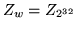. Vectors are indexed from zero, so a vector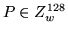of 128 32-bit numbers will be indexed as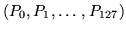. The symbol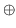represents bitwise exclusive OR; where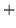appears in the figures with a square box around it, it represents addition in the ring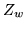. Least significant and lowest indexed bytes and words appear leftmost and uppermost in the figures.

Note that some details that would be needed to build a specification of Mercy-based file encryption sufficient for interoperability, such as byte ordering within words, are omitted since they are irrelevant for cryptanalytic purposes.

## T box

The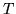box (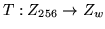, Figure 1) is a key-dependent mapping of bytes onto words.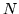represents multiplicative inverses in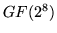with polynomial base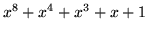except that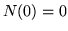.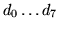are key dependent bijective affine mappings on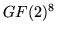.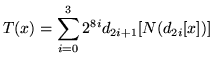## Operation M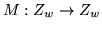(Figure 2) is drawn from David Wheeler's stream cipher WAKE ; it's a simple, key-dependent mapping on 32-bit words. The most significant byte of the input word is looked up in thebox, and the output XORred with the other three bytes shifted up eight bits; the construction of thebox ensures that this mapping is bijective.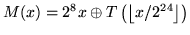## Q state machine

The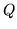state machine (Figure 3) maps a four-word initial state and one word input onto a four-word final state and one word output (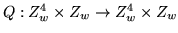) using taps from a linear feedback shift register and a nonlinear mixing function.

## F function

The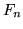function (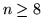; Figure 4) accepts a 128-bit spice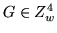and a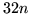-bit input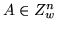and generates a-bit output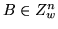(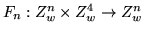).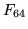(usually just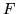) is the F function for the Feistel rounds. Here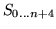represents successive 128-bit states of a state machine;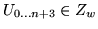are the successive 32-bit inputs to the state machine, and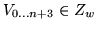are the outputs.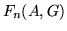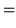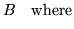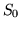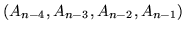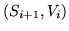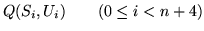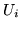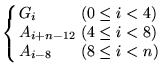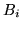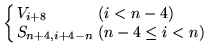## Round structure

Mercy uses a six round Feistel structure (Figure 5) with partial pre- and post-whitening; unusually, the final swap is not omitted. The spice(usually the sector number) goes through a spice scheduling'' procedure, analogous with key scheduling, through which the spice material''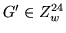is generated based on the input spice, using the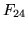variant of thefunction; this forms six 128-bit round spices''. Spice scheduling uses a dummy input to the F function; for this a vector of incrementing bytes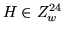is used.represents the plaintext,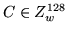the ciphertext, and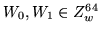the whitening values. We describe decryption below; since Mercy is a straightforward Feistel cipher encryption follows in the straightforward way.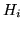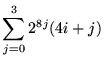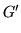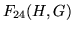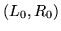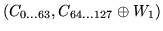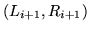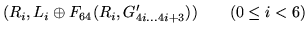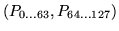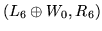## Key schedule

The key is used to seed a CPRNG from which key material is drawn;  is used in the sample implementation (after discarding 256 bytes of output), and is convenient since it's small and byte oriented, but any strong CPRNG will serve. Then the procedure in Figure 6 generates the substitutions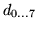and the 2048-bit whitening values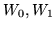.

An expected 10.6 random bytes will be drawn for each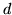. Oncehave been determined, a 1k table representing thebox can be generated. During normal use 1536 bytes of key-dependent tables are used.Next: Design of Mercy Up: Mercy: a fast large Previous: Mercy design goals

mercy@paul.cluefactory.org.uk